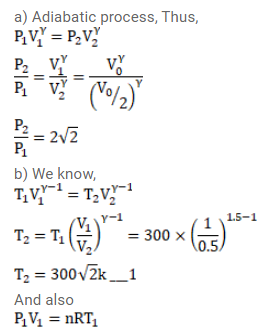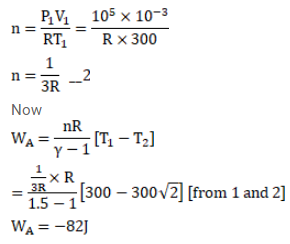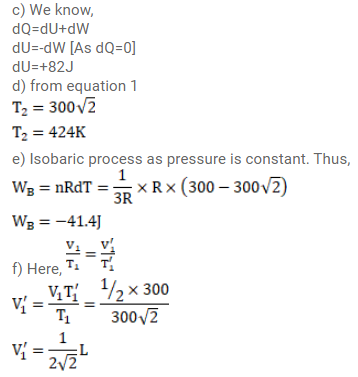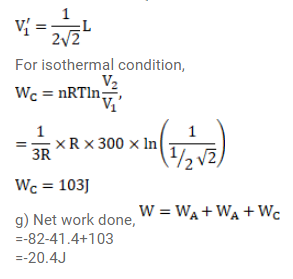# 1 liter of an ideal gas (γ=1.5) at 300 K is suddenly compressed`
Question:

1 liter of an ideal gas $(\gamma=1.5)$ at $300 \mathrm{~K}$ is suddenly compressed to half its original volume.

(a) Find the ratio of the final pressure to the initial pressure.

(b) If the original pressure is $100 \mathrm{kPa}$, find the work done by the gas in the process.

(c) What is the change in internal energy?

(d) What is the final temperature?

(e) The gas is now cooled to $300 \mathrm{~K}$ keeping its pressure constant. Calculate the work done during the process.

(f) The gas is now expanded isothermally to achieve its original volume of 1 liter. Calculate the work done by the gas.

(g) Calculate the total work done in the cycle.

Solution: